# What is the Mole?

The mole is just a number like a dozen, a hundred, or a million. By definition, a mole is the number of atoms in a 12-g sample of 12C isotope which happens to be 6.022 x 1023 atoms. This is called Avogadro’s number (NA = 6.022 x 1023).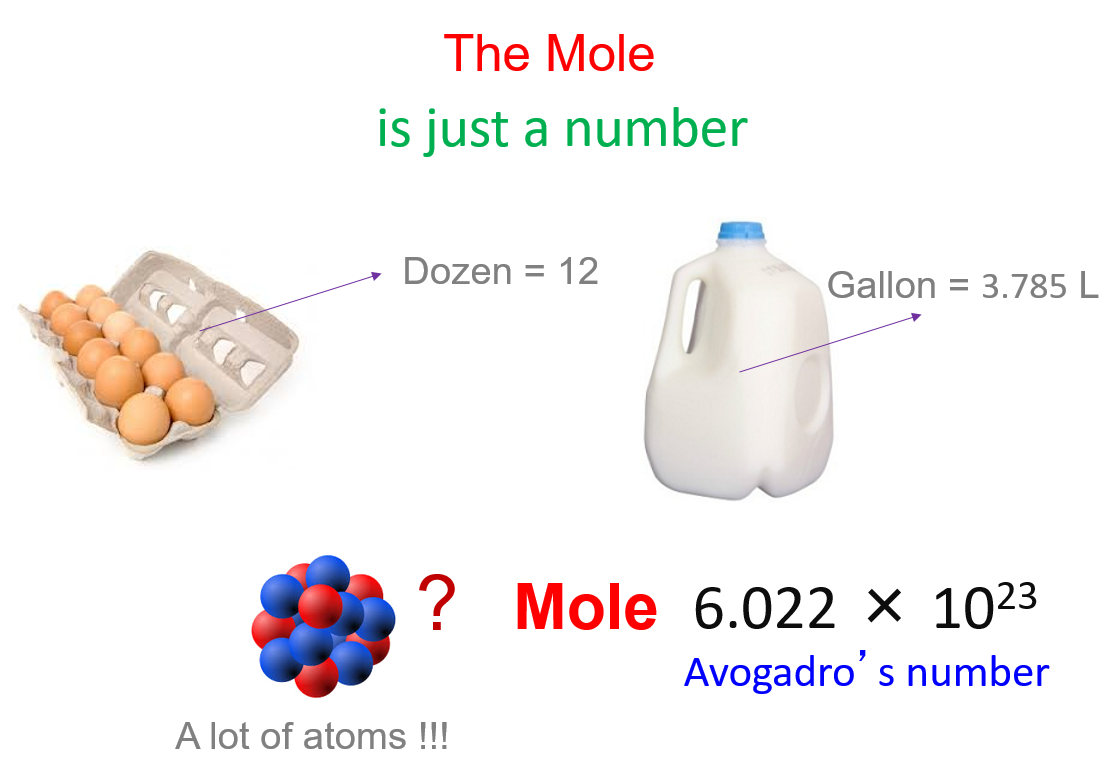One mole of any element contains 6.022 x 1023 of its atoms, and a mole of a compound contains 6.022 x 1023 molecules, ions-ions, and in general, 1 mol = 6.022 x 1023 particles.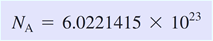# Molar Mass

The mass of one mole of atoms/molecules/ions is called its molar mass (M) expressed in g/mol.

Numerically, the molar mass is equal to the atomic mass of a given atom or a molecule, so we can look up the molar mass of an element in the periodic table.

For example, we can say that the mass of Cu is 63.55 amu or 63.55 g/mol. The molecular mass of water is 18.0 amu, and the molar mass is 18.0 g/mol.

For the molar mass, keeping one decimal is usually an acceptable approximation, and for the Avogadro’s number, you can use 6.02 x 1023.

# Calculating Moles from the Mass

To calculate the moles from a given mass (m), the molar mass of the component is used. The given mass is the mass of the sample, and it can be any number, for example, we can have 10 g of salt, 15 g, or 100 g. The molar mass, on the other hand, is a constant number for a given atom or a molecule as it is for a specific amount of it.

So, to calculate the moles from a given mass, we make a conversion factor correlating 1 mole with the molar of the given component.

For example, how many moles of sulfur are there in a 16.2 g sample?

The conversion factors are:

$\frac{{{\rm{1}}\;{\rm{mol}}\;{\rm{S}}}}{{{\rm{32}}{\rm{.1}}\;{\rm{g}}\,{\rm{S}}}}\;\,and\,\,\frac{{{\rm{32}}{\rm{.1}}\;{\rm{g}}\,{\rm{S}}}}{{{\rm{1}}\;{\rm{mol}}\;{\rm{S}}}}$

These two simply indicate that 1 mol of S weighs 32.1 g, which is the molar mass of the sulfur, and we can find it in the periodic table.

To calculate the number of moles, write the given mass and multiply by the conversion factor that has the mass in the denominator so that they can be canceled: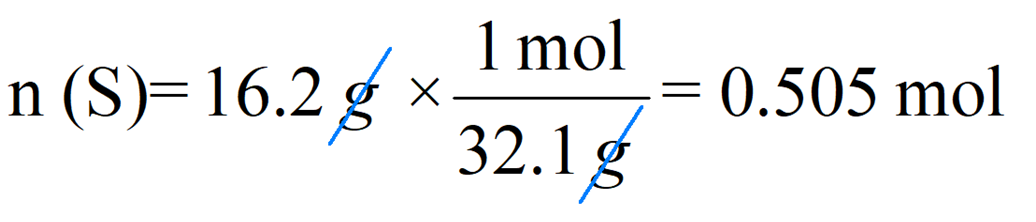You can also do this by using the formula: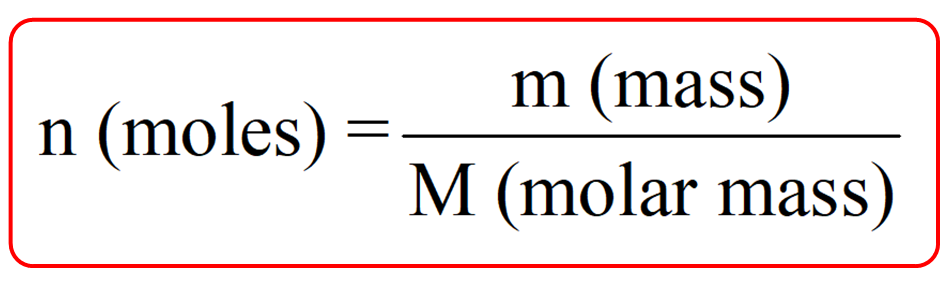So, for 13.2 g of sulfur, we’d have: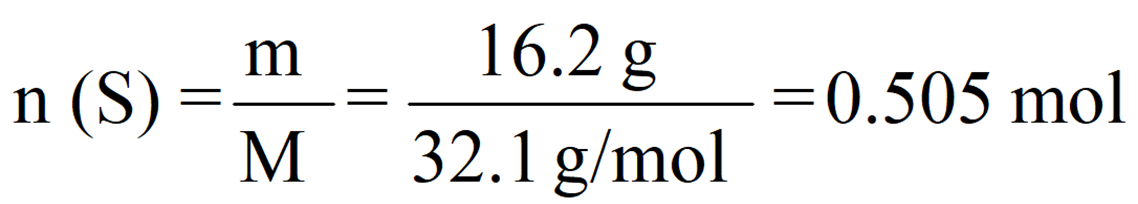Notice that the exact answer in the calculator is 0.5046728 and we round it off to three significant figures because both initial numbers contain three significant figures.

# Mass from the Moles

If the question asks us to calculate the mass from the moles, we will need to use the other conversion factor so that the moles now can be canceled.

For example, how many moles of carbon are there in a 0.0480 mol sample?

The molar mass of carbon is 12.0 g/mol, so the conversion factors are:

$\frac{{{\rm{1}}\;{\rm{mol}}\;{\rm{C}}}}{{{\rm{12}}{\rm{.0}}\;{\rm{g}}\,{\rm{S}}}}\;\,and\,\,\frac{{{\rm{12}}{\rm{.0}}\;{\rm{g}}\,{\rm{C}}}}{{{\rm{1}}\;{\rm{mol}}\;{\rm{C}}}}$

Using the second conversion factor, we can calculate the mass as:

${\rm{m}}\;{\rm{(C)}}\,{\rm{ = }}\;{\rm{0}}{\rm{.0480}}\;\cancel{{{\rm{mol}}\,{\rm{C}}}}\,{\rm{ \times }}\,\frac{{{\rm{12}}{\rm{.0}}\;{\rm{g}}\,{\rm{C}}}}{{{\rm{1}}\;\cancel{{{\rm{mol}}\;{\rm{C}}}}}}\;{\rm{ = }}\;{\rm{0}}{\rm{.576}}\,{\rm{g}}$

# Number of Atoms from the Moles

Whether it is the number of atoms, molecules, or ions, use Avogadro’s number to calculate it from the moles.

For example, calculate the number of iron atoms in a 3.5-mole sample.

This time, we are going to use a conversion factor correlating 1 mole and the Avogadro’s number. Since 1 mole = 6.022 x 1023, we can write these two conversion factors:

$\frac{{{\rm{1}}\;{\rm{mol}}\;{\rm{Fe}}}}{{{\rm{6}}{\rm{.022}}\;{\rm{ \times }}\,{\rm{1}}{{\rm{0}}^{{\rm{23}}}}\,{\rm{Fe}}\;{\rm{atoms}}}}\;\;\;\,and\;\;\,\,\frac{{{\rm{6}}{\rm{.022}}\;{\rm{ \times }}\,{\rm{1}}{{\rm{0}}^{{\rm{23}}}}\,{\rm{Fe}}\;{\rm{atoms}}}}{{{\rm{1}}\;{\rm{mol}}\;{\rm{Fe}}}}$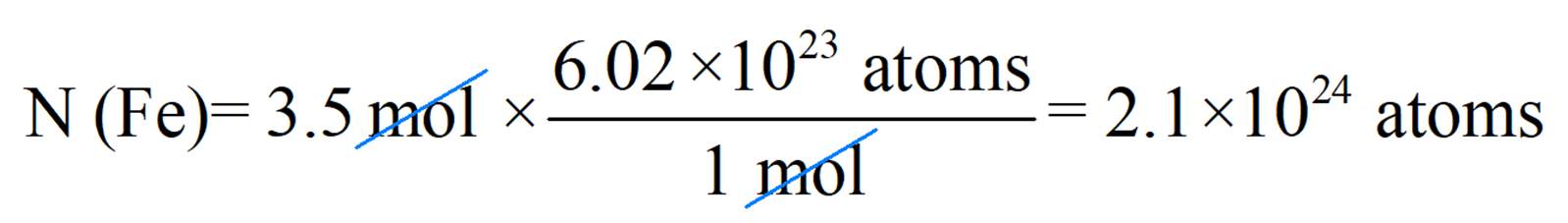# Number of Atoms from the Mass

This is going to be one additional step compared to the conversion from the number of moles. There are two steps combined in this conversion and the plan is to first convert the mass to moles and then to the number of atoms using NA: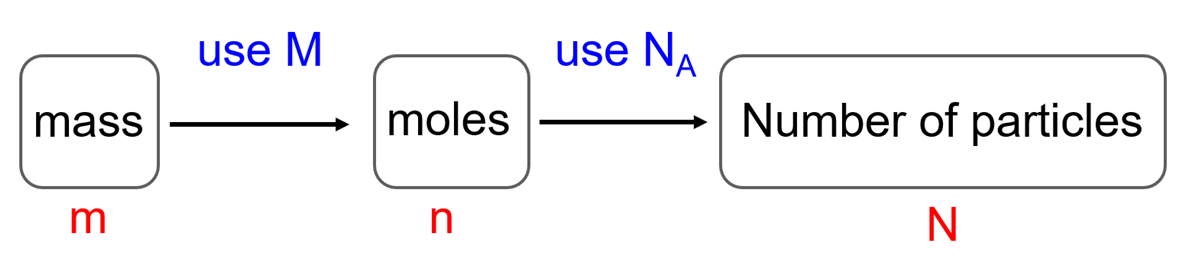For example, calculate the number of copper atoms in its 44.52 g sample.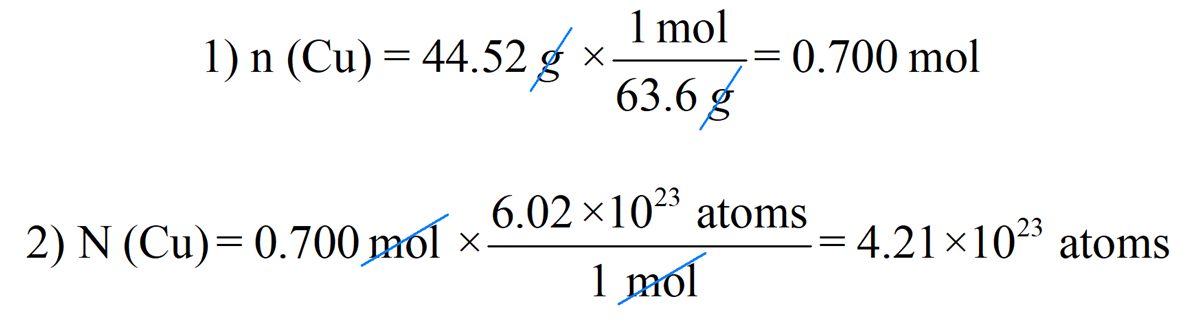You can also do this in a one-step conversion by combining these two together: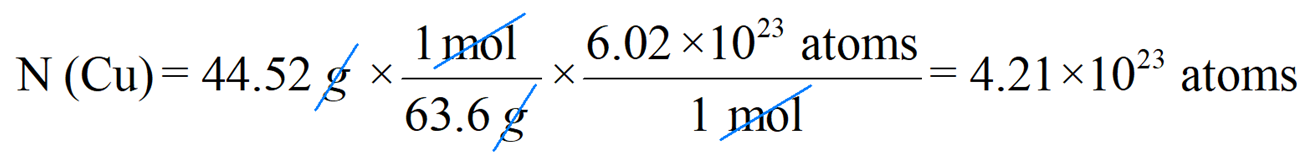# Number of Atoms in a Molecule

This adds another step because there may be more than one atom in a given molecule. In this case, to find the number of atoms/ions in a molecule, multiply the number of molecules by the subscript of that atom.

For example, how many Na ions are there in a 658 g sample of Na2CO3?

In each unit of Na2CO3, there are 2 Na atoms, so we are going to determine the number of Na2CO3 molecules and multiply it by two.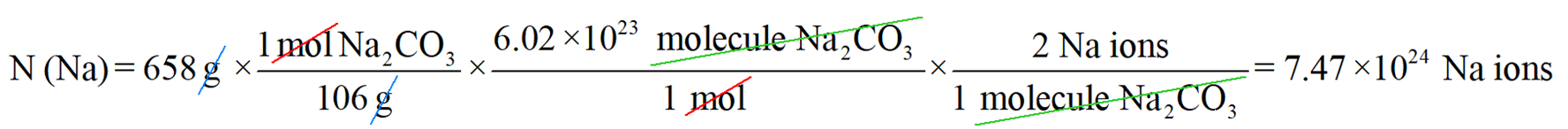Let’s also break this down and do each step of the calculation separately.

Step 1. In the first step, determine the number of moles:

${\rm{n}}\;{\rm{(N}}{{\rm{a}}_{\rm{2}}}{\rm{C}}{{\rm{O}}_{\rm{3}}}{\rm{)}}\,{\rm{ = }}\;{\rm{658}}\cancel{{\rm{g}}}\;{\rm{ \times }}\,\frac{{{\rm{1}}\cancel{{{\rm{mol}}}}{\rm{N}}{{\rm{a}}_{\rm{2}}}{\rm{C}}{{\rm{O}}_{\rm{3}}}}}{{{\rm{106}}\cancel{{\rm{g}}}}}\;{\rm{ = }}\;{\rm{6}}{\rm{.20}}\;{\rm{mol}}\;$

Step 2. Calculate the number of molecules in 6.20 moles of Na2CO3:

${\rm{N}}\;{\rm{(N}}{{\rm{a}}_{\rm{2}}}{\rm{C}}{{\rm{O}}_{\rm{3}}}{\rm{)}}\,{\rm{ = }}\;{\rm{6}}{\rm{.20}}\cancel{{{\rm{mol}}}}\;{\rm{ \times }}\,\frac{{{\rm{6}}{\rm{.02}}\;{\rm{ \times }}\,{\rm{1}}{{\rm{0}}^{{\rm{23}}}}\;{\rm{molecule}}\;{\rm{N}}{{\rm{a}}_{\rm{2}}}{\rm{C}}{{\rm{O}}_{\rm{3}}}}}{{{\rm{1}}\cancel{{{\rm{mol}}}}\;{\rm{N}}{{\rm{a}}_{\rm{2}}}{\rm{C}}{{\rm{O}}_{\rm{3}}}}}\; = \;3.73\;{\rm{ \times }}\,{\rm{1}}{{\rm{0}}^{{\rm{24}}}}\;\;\;$

Step 3. In the last step, we recognize that every molecule Na2CO3 has two sodium atoms, and therefore, we multiply the number of molecules by two to determine the number of Na atoms:

${\rm{N}}\;{\rm{(Na)}}\,{\rm{ = }}\;3.73\cancel{{{\rm{ \times }}\,{\rm{1}}{{\rm{0}}^{{\rm{24}}}}{\rm{molecule}}\;{\rm{N}}{{\rm{a}}_{\rm{2}}}{\rm{C}}{{\rm{O}}_{\rm{3}}}}}\;{\rm{ \times }}\,\frac{{{\rm{2}}\;{\rm{Na}}\;{\rm{ions}}}}{{\cancel{{{\rm{1}}\;{\rm{molecule}}\;{\rm{N}}{{\rm{a}}_{\rm{2}}}{\rm{C}}{{\rm{O}}_{\rm{3}}}}}}}\;{\rm{ = }}\;{\rm{7}}{\rm{.46}}\;{\rm{ \times }}\,{\rm{1}}{{\rm{0}}^{{\rm{24}}}}\;{\rm{Na}}\;{\rm{ions}}$

The little inconsistency in the final answer is due to rounding off the intermediate numbers. I do it here mainly to save space, but you can keep the numbers in the calculator and round them off at the end. This is the accurate way of rounding off to the correct number of significant figures in multistep calculations.

Note: Although Na2CO3 is an ionic compound and sodium is present in an ionic form, it is a habitual way of referring to an element in these types of calculations.

Check Also

1.

## Calculating Moles from Mass

Determine the number of moles in 59.7 grams of Al.

This content is available to registered users only. Click here to Register! By joining Chemistry Steps, you will gain instant access to the answers and solutions for all the Practice Problems and the powerful set of General Chemistry 1 and 2 Summary Study Guides.
Solution
This content is available to registered users only. Click here to Register! By joining Chemistry Steps, you will gain instant access to the answers and solutions for all the Practice Problems and the powerful set of General Chemistry 1 and 2 Summary Study Guides.
2.

Determine the number of moles in 2.41 grams of FeO.

This content is available to registered users only. Click here to Register! By joining Chemistry Steps, you will gain instant access to the answers and solutions for all the Practice Problems and the powerful set of General Chemistry 1 and 2 Summary Study Guides.
Solution
This content is available to registered users only. Click here to Register! By joining Chemistry Steps, you will gain instant access to the answers and solutions for all the Practice Problems and the powerful set of General Chemistry 1 and 2 Summary Study Guides.
3.

Calculate the number of moles in 0.647 grams of Al2O3.

This content is available to registered users only. Click here to Register! By joining Chemistry Steps, you will gain instant access to the answers and solutions for all the Practice Problems and the powerful set of General Chemistry 1 and 2 Summary Study Guides.
Solution
This content is available to registered users only. Click here to Register! By joining Chemistry Steps, you will gain instant access to the answers and solutions for all the Practice Problems and the powerful set of General Chemistry 1 and 2 Summary Study Guides.
4.

Determine the number of moles in 3.56 grams of Mg(OH)2.

This content is available to registered users only. Click here to Register! By joining Chemistry Steps, you will gain instant access to the answers and solutions for all the Practice Problems and the powerful set of General Chemistry 1 and 2 Summary Study Guides.
Solution
This content is available to registered users only. Click here to Register! By joining Chemistry Steps, you will gain instant access to the answers and solutions for all the Practice Problems and the powerful set of General Chemistry 1 and 2 Summary Study Guides.
5.

Determine the number of moles in 0.385 grams of N2O3.

This content is available to registered users only. Click here to Register! By joining Chemistry Steps, you will gain instant access to the answers and solutions for all the Practice Problems and the powerful set of General Chemistry 1 and 2 Summary Study Guides.
Solution
This content is available to registered users only. Click here to Register! By joining Chemistry Steps, you will gain instant access to the answers and solutions for all the Practice Problems and the powerful set of General Chemistry 1 and 2 Summary Study Guides.
6.

Determine the number of moles in 165 grams of CaSO4.

This content is available to registered users only. Click here to Register! By joining Chemistry Steps, you will gain instant access to the answers and solutions for all the Practice Problems and the powerful set of General Chemistry 1 and 2 Summary Study Guides.
Solution
This content is available to registered users only. Click here to Register! By joining Chemistry Steps, you will gain instant access to the answers and solutions for all the Practice Problems and the powerful set of General Chemistry 1 and 2 Summary Study Guides.
7.

Calculate the molar mass of N2O4 and determine how many moles of it are in a 23.9 g sample.

This content is available to registered users only. Click here to Register! By joining Chemistry Steps, you will gain instant access to the answers and solutions for all the Practice Problems and the powerful set of General Chemistry 1 and 2 Summary Study Guides.
Solution
This content is available to registered users only. Click here to Register! By joining Chemistry Steps, you will gain instant access to the answers and solutions for all the Practice Problems and the powerful set of General Chemistry 1 and 2 Summary Study Guides.
8.

Calculate the number of moles in 165 grams of C3H6O.

This content is available to registered users only. Click here to Register! By joining Chemistry Steps, you will gain instant access to the answers and solutions for all the Practice Problems and the powerful set of General Chemistry 1 and 2 Summary Study Guides.
Solution
This content is available to registered users only. Click here to Register! By joining Chemistry Steps, you will gain instant access to the answers and solutions for all the Practice Problems and the powerful set of General Chemistry 1 and 2 Summary Study Guides.
9.

Determine the number of moles in 452 grams of Co(NO3)3.

This content is available to registered users only. Click here to Register! By joining Chemistry Steps, you will gain instant access to the answers and solutions for all the Practice Problems and the powerful set of General Chemistry 1 and 2 Summary Study Guides.
Solution
This content is available to registered users only. Click here to Register! By joining Chemistry Steps, you will gain instant access to the answers and solutions for all the Practice Problems and the powerful set of General Chemistry 1 and 2 Summary Study Guides.
10.

## Calculating Mass from Moles

Calculate the mass in grams of 0.598 moles of Fe.

This content is available to registered users only. Click here to Register! By joining Chemistry Steps, you will gain instant access to the answers and solutions for all the Practice Problems and the powerful set of General Chemistry 1 and 2 Summary Study Guides.
Solution
This content is available to registered users only. Click here to Register! By joining Chemistry Steps, you will gain instant access to the answers and solutions for all the Practice Problems and the powerful set of General Chemistry 1 and 2 Summary Study Guides.
11.

Calculate the mass in grams of 0.168 moles of NO.

This content is available to registered users only. Click here to Register! By joining Chemistry Steps, you will gain instant access to the answers and solutions for all the Practice Problems and the powerful set of General Chemistry 1 and 2 Summary Study Guides.
Solution
This content is available to registered users only. Click here to Register! By joining Chemistry Steps, you will gain instant access to the answers and solutions for all the Practice Problems and the powerful set of General Chemistry 1 and 2 Summary Study Guides.
12.

Calculate the mass in grams of 0.987 moles of (NH4)2S.

This content is available to registered users only. Click here to Register! By joining Chemistry Steps, you will gain instant access to the answers and solutions for all the Practice Problems and the powerful set of General Chemistry 1 and 2 Summary Study Guides.
Solution
This content is available to registered users only. Click here to Register! By joining Chemistry Steps, you will gain instant access to the answers and solutions for all the Practice Problems and the powerful set of General Chemistry 1 and 2 Summary Study Guides.
13.

Calculate the mass in grams of 6.81 moles of Al2(SO4)3.

This content is available to registered users only. Click here to Register! By joining Chemistry Steps, you will gain instant access to the answers and solutions for all the Practice Problems and the powerful set of General Chemistry 1 and 2 Summary Study Guides.
Solution
This content is available to registered users only. Click here to Register! By joining Chemistry Steps, you will gain instant access to the answers and solutions for all the Practice Problems and the powerful set of General Chemistry 1 and 2 Summary Study Guides.
14.

Calculate the mass in grams of 2.64 moles of methanol, CH3OH.

This content is available to registered users only. Click here to Register! By joining Chemistry Steps, you will gain instant access to the answers and solutions for all the Practice Problems and the powerful set of General Chemistry 1 and 2 Summary Study Guides.
Solution
This content is available to registered users only. Click here to Register! By joining Chemistry Steps, you will gain instant access to the answers and solutions for all the Practice Problems and the powerful set of General Chemistry 1 and 2 Summary Study Guides.
15.

Calculate the mass in grams of 9.42 moles of NiCl2·6H2O.

This content is available to registered users only. Click here to Register! By joining Chemistry Steps, you will gain instant access to the answers and solutions for all the Practice Problems and the powerful set of General Chemistry 1 and 2 Summary Study Guides.
Solution
This content is available to registered users only. Click here to Register! By joining Chemistry Steps, you will gain instant access to the answers and solutions for all the Practice Problems and the powerful set of General Chemistry 1 and 2 Summary Study Guides.
16.

## Calculating the Number of Molecules from the Moles

How many molecules are there in a 0.487 mol sample of PCl5?

This content is available to registered users only. Click here to Register! By joining Chemistry Steps, you will gain instant access to the answers and solutions for all the Practice Problems and the powerful set of General Chemistry 1 and 2 Summary Study Guides.
Solution
This content is available to registered users only. Click here to Register! By joining Chemistry Steps, you will gain instant access to the answers and solutions for all the Practice Problems and the powerful set of General Chemistry 1 and 2 Summary Study Guides.
17.

How many molecules (formula units) are there in a 5.84 mol sample of Na2SO3.

This content is available to registered users only. Click here to Register! By joining Chemistry Steps, you will gain instant access to the answers and solutions for all the Practice Problems and the powerful set of General Chemistry 1 and 2 Summary Study Guides.
Solution
This content is available to registered users only. Click here to Register! By joining Chemistry Steps, you will gain instant access to the answers and solutions for all the Practice Problems and the powerful set of General Chemistry 1 and 2 Summary Study Guides.
18.

How many molecules of sucrose, C12H22O11 are there in a 0.684 mol sample?

This content is available to registered users only. Click here to Register! By joining Chemistry Steps, you will gain instant access to the answers and solutions for all the Practice Problems and the powerful set of General Chemistry 1 and 2 Summary Study Guides.
Solution
This content is available to registered users only. Click here to Register! By joining Chemistry Steps, you will gain instant access to the answers and solutions for all the Practice Problems and the powerful set of General Chemistry 1 and 2 Summary Study Guides.
19.

Calculate the number of molecules in a 3.25-mol sample of propane, C3H8.

This content is available to registered users only. Click here to Register! By joining Chemistry Steps, you will gain instant access to the answers and solutions for all the Practice Problems and the powerful set of General Chemistry 1 and 2 Summary Study Guides.
Solution
This content is available to registered users only. Click here to Register! By joining Chemistry Steps, you will gain instant access to the answers and solutions for all the Practice Problems and the powerful set of General Chemistry 1 and 2 Summary Study Guides.
20.

How many moles is 5.80 x 1025 molecules of POCl3?

This content is available to registered users only. Click here to Register! By joining Chemistry Steps, you will gain instant access to the answers and solutions for all the Practice Problems and the powerful set of General Chemistry 1 and 2 Summary Study Guides.
Solution
This content is available to registered users only. Click here to Register! By joining Chemistry Steps, you will gain instant access to the answers and solutions for all the Practice Problems and the powerful set of General Chemistry 1 and 2 Summary Study Guides.
21.

## Calculating the Number of Molecules from the Mass

How many molecules are there in a 5.12-g sample of K2O?

This content is available to registered users only. Click here to Register! By joining Chemistry Steps, you will gain instant access to the answers and solutions for all the Practice Problems and the powerful set of General Chemistry 1 and 2 Summary Study Guides.
Solution
This content is available to registered users only. Click here to Register! By joining Chemistry Steps, you will gain instant access to the answers and solutions for all the Practice Problems and the powerful set of General Chemistry 1 and 2 Summary Study Guides.
22.

How many molecules of glucose, C6H12O6 are there in a 35.0 g sample?

This content is available to registered users only. Click here to Register! By joining Chemistry Steps, you will gain instant access to the answers and solutions for all the Practice Problems and the powerful set of General Chemistry 1 and 2 Summary Study Guides.
Solution
This content is available to registered users only. Click here to Register! By joining Chemistry Steps, you will gain instant access to the answers and solutions for all the Practice Problems and the powerful set of General Chemistry 1 and 2 Summary Study Guides.
23.

Calculate the number of molecules of butane, C4H10, in its 2.40-gram sample.

This content is available to registered users only. Click here to Register! By joining Chemistry Steps, you will gain instant access to the answers and solutions for all the Practice Problems and the powerful set of General Chemistry 1 and 2 Summary Study Guides.
Solution
This content is available to registered users only. Click here to Register! By joining Chemistry Steps, you will gain instant access to the answers and solutions for all the Practice Problems and the powerful set of General Chemistry 1 and 2 Summary Study Guides.
24.

How many Ethylene, C2H4 molecules are present in a 46.2 g sample? The molar mass of C2H4 is 28.1 g/mol.

This content is available to registered users only. Click here to Register! By joining Chemistry Steps, you will gain instant access to the answers and solutions for all the Practice Problems and the powerful set of General Chemistry 1 and 2 Summary Study Guides.
Solution
This content is available to registered users only. Click here to Register! By joining Chemistry Steps, you will gain instant access to the answers and solutions for all the Practice Problems and the powerful set of General Chemistry 1 and 2 Summary Study Guides.
25.

## Calculating the Number of Atoms

Calculate the number of atoms in a 2.56-g sample of Ca.

This content is available to registered users only. Click here to Register! By joining Chemistry Steps, you will gain instant access to the answers and solutions for all the Practice Problems and the powerful set of General Chemistry 1 and 2 Summary Study Guides.
Solution
This content is available to registered users only. Click here to Register! By joining Chemistry Steps, you will gain instant access to the answers and solutions for all the Practice Problems and the powerful set of General Chemistry 1 and 2 Summary Study Guides.
26.

How many carbon atoms are there in a 0.590 mol sample of CCl4.

This content is available to registered users only. Click here to Register! By joining Chemistry Steps, you will gain instant access to the answers and solutions for all the Practice Problems and the powerful set of General Chemistry 1 and 2 Summary Study Guides.
Solution
This content is available to registered users only. Click here to Register! By joining Chemistry Steps, you will gain instant access to the answers and solutions for all the Practice Problems and the powerful set of General Chemistry 1 and 2 Summary Study Guides.
27.

How many carbon atoms are there in a 0.964 mol sample of C2H6.

This content is available to registered users only. Click here to Register! By joining Chemistry Steps, you will gain instant access to the answers and solutions for all the Practice Problems and the powerful set of General Chemistry 1 and 2 Summary Study Guides.
Solution
This content is available to registered users only. Click here to Register! By joining Chemistry Steps, you will gain instant access to the answers and solutions for all the Practice Problems and the powerful set of General Chemistry 1 and 2 Summary Study Guides.
28.

Which sample contains more Cl atoms: a) 1.25 moles of CH2Cl2 b) 2.15 moles of CH3Cl

This content is available to registered users only. Click here to Register! By joining Chemistry Steps, you will gain instant access to the answers and solutions for all the Practice Problems and the powerful set of General Chemistry 1 and 2 Summary Study Guides.
Solution
This content is available to registered users only. Click here to Register! By joining Chemistry Steps, you will gain instant access to the answers and solutions for all the Practice Problems and the powerful set of General Chemistry 1 and 2 Summary Study Guides.
29.

The molecular formula of morphine is C17H19NO3. How many carbon atoms are in a 34.7-gram sample of morphine?

This content is available to registered users only. Click here to Register! By joining Chemistry Steps, you will gain instant access to the answers and solutions for all the Practice Problems and the powerful set of General Chemistry 1 and 2 Summary Study Guides.
Solution
This content is available to registered users only. Click here to Register! By joining Chemistry Steps, you will gain instant access to the answers and solutions for all the Practice Problems and the powerful set of General Chemistry 1 and 2 Summary Study Guides.
30.

Isopropyl alcohol, also known as isopropanol, has found a widespread application in the preparation of pharmaceutical products. Answer the following questions considering that the molecular formula of isopropanol is C3H8O.

a) How many moles of C3H8O are contained in a 12.0 g sample of the alcohol?
b) How many molecules of C3H8O are contained in a 12.0 g sample of the alcohol?
c) How many atoms of oxygen are contained in a 12.0 g sample of the isopropyl alcohol (C3H8O)?
d) How many atoms of carbon are contained in a 12.0 g sample of the isopropyl alcohol (C3H8O)?

a)